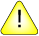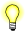This version of this document is no longer maintained. For the latest documentation, see http://www.qnx.com/developers/docs.

# initstate()

Initialize a pseudo-random number generator

## Synopsis:

```#include <stdlib.h>

char* initstate( unsigned int seed,
char* state,
size_t size );```

## Arguments:

seed
A starting point for the random-number sequence. This lets you restart the sequence at the same point.
state
The state array that you want to initialize.
size
The size, in bytes, of the state array; see below.

## Library:

libc

Use the -l c option to qcc to link against this library. This library is usually included automatically.This function is in libc.a, but not in libc.so (in order to save space).

## Description:

The initstate() initializes the given state array for future use when generating pseudo-random numbers.

This function uses the size argument to determine what type of random-number generator to use; the larger the state array, the more random the numbers. Values for the amount of state information are 8, 32, 64, 128, and 256 bytes. Other values greater than 8 bytes are rounded down to the nearest one of these values. For values smaller than 8, random() uses a simple linear congruential random number generator.

Use this function in conjunction with the following:

random()
Generate a pseudo-random number using a default state.
setstate()
Specify the state of the pseudo-random number generator.
srandom()
Set the seed used by the pseudo-random number generator.

If you haven't called initstate(), random() behaves as though you had called initstate() with a seed of 1 and a size of 128.

After initialization, you can restart a state array at a different point in one of these ways:

• Call initstate() with the desired seed, state array, and size of the array.
• Call setstate() with the desired state, then call srandom() with the desired seed. The advantage of using both of these functions is that the size of the state array doesn't have to be saved once it's initialized.

## Returns:

A pointer to the previous state array, or NULL if an error occurred.

## Examples:

```#include <stdlib.h>
#include <stdio.h>
#include <time.h>

static char state1;

int main() {
initstate( time(NULL), state1, sizeof(state1));
setstate(state1);
printf("%d0\n", random());
return EXIT_SUCCESS;
}```

## Classification:

POSIX 1003.1 XSI

Safety:
Cancellation point Yes
Interrupt handler No
Signal handler No